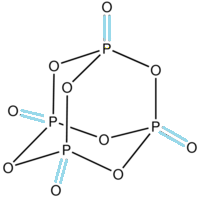Courses
Courses for Kids
Free study material
Free LIVE classes
MoreLIVE
Join Vedantu’s FREE Mastercalss

# In ${{\text{P}}_{4}}{{\text{O}}_{10}}$, the number of oxygen atoms attached to each phosphorus atom is:A. 2B. 3C. 4D. 5Verified
333.9k+ views
Hint: ${{\text{P}}_{4}}{{\text{O}}_{10}}$ is a molecular formula of pentoxide which consists of 4 molecules of phosphorus and 10 molecules of oxygen. The phosphorus can make a maximum of 5 bonds with other molecules whereas oxygen can make a maximum of 2 bonds with other molecules.

-To count the number of bonds between oxygen and phosphorus we first have to draw the structure of phosphorus pentoxide.
-The structure is given below:-As we can see in the structure of phosphorus pentoxide there are a total 4 oxygens which are highlighted by blue colour and make double bonds only with a single phosphorus atom.
-The double-bonded oxygen has a hybridisation of $\text{s}{{\text{p}}^{2}}$
-Whereas 6 oxygen atoms are attached to the two phosphorus atoms each with a single bond in which the hybridisation of oxygen atom is $\text{s}{{\text{p}}^{3}}$.
So, option C. is the correct answer.
-The structure of phosphorus pentoxide forms a hexagonal lattice and is held together by the Vander Waal forces.
-The structure consists of at least four polymorphs.
Note: The preparation of phosphorus pentoxide is done when tetraphosphorus reacts with oxygen molecules: ${{\text{P}}_{4}}\text{ + 5}{{\text{O}}_{2}}\text{ }\to \text{ }{{\text{P}}_{4}}{{\text{O}}_{10}}$. The compound is used as a dehydrating agent and is white in appearance. The name of the compound is named so because it is the empirical formula of ${{\text{P}}_{4}}{{\text{O}}_{5}}$.
Last updated date: 19th Sep 2023
Total views: 333.9k
Views today: 8.33k